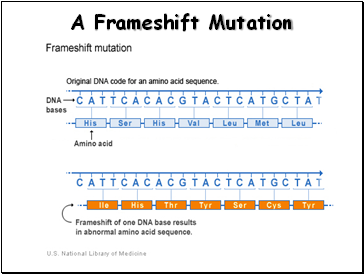MutationsPage 1

WATCH ALL SLIDES

Slide 1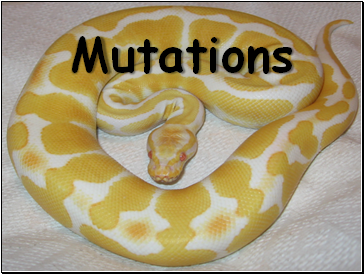Mutations

Slide 2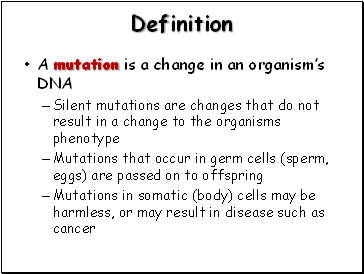Definition

A mutation is a change in an organism’s DNA

Silent mutations are changes that do not result in a change to the organisms phenotype

Mutations that occur in germ cells (sperm, eggs) are passed on to offspring

Mutations in somatic (body) cells may be harmless, or may result in disease such as cancer

Slide 3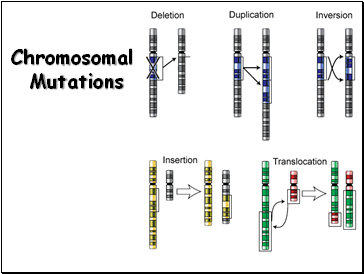Chromosomal Mutations

Slide 4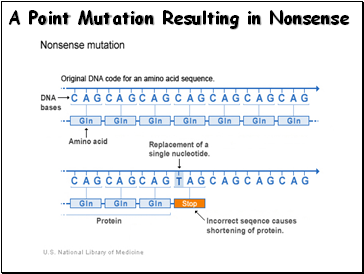A Point Mutation Resulting in Nonsense

Slide 5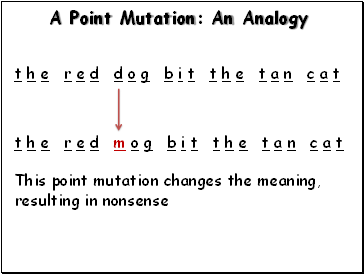A Point Mutation: An Analogy

t h e r e d d o g b i t t h e t a n c a t

t h e r e d m o g b i t t h e t a n c a t

This point mutation changes the meaning,

resulting in nonsense

Slide 6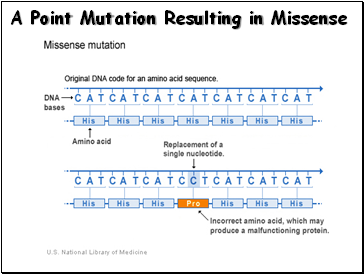A Point Mutation Resulting in Missense

Slide 7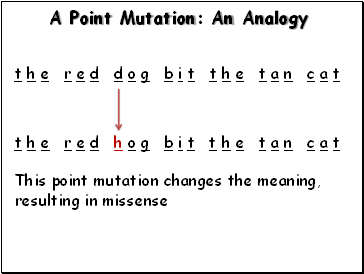A Point Mutation: An Analogy

t h e r e d d o g b i t t h e t a n c a t

t h e r e d h o g b i t t h e t a n c a t

This point mutation changes the meaning,

resulting in missense

Slide 8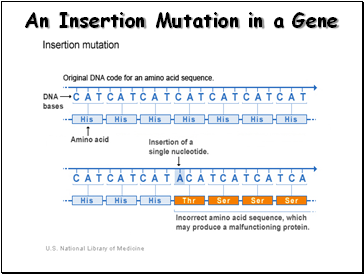An Insertion Mutation in a Gene

Slide 9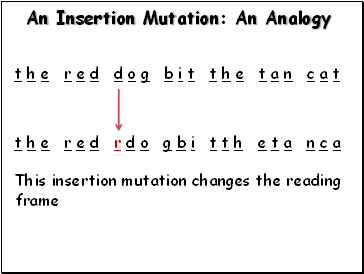An Insertion Mutation: An Analogy

t h e r e d d o g b i t t h e t a n c a t

t h e r e d r d o g b i t t h e t a n c a

This insertion mutation changes the reading

frame

Slide 10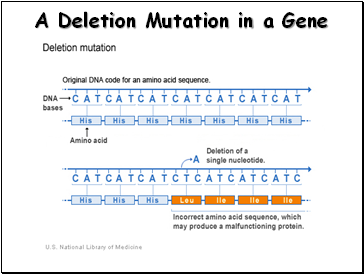A Deletion Mutation in a Gene

Slide 11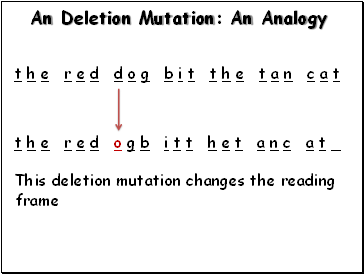An Deletion Mutation: An Analogy

t h e r e d d o g b i t t h e t a n c a t

t h e r e d o g b i t t h e t a n c a t _

This deletion mutation changes the reading

frame

Slide 12